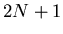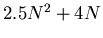Next: Application example - echo Up: number9 Previous: The LMS adaptive filter

The RLS filter

The Recursive Least Squares (RLS) filter is in theory a better filter than the LMS filter, but it is not used as often as it could be because it requires more computational resources (The LMS filter requiresoperations per filter update, whereas the RLS filter requires). It has been sucessfully used in system identification problems and in time series analysis where its real-time performance is not an issue.

There are several forms of the RLS filter, but all of them are similar to the Kalman filter in that a covariance is calculated along with the regular filter output. This covariance is then used to calculate a new filter gain.

Listing 1 shows a Forth implementation of the square-root form of the filter. This form of the filter calculates the square root of the covariance instead of the covariance directly in order to improve the numerical stability of the filter. The filter has two tunable parameters, sigma is the square root of the variance of the input data, lambda is a weighting coefficient that controls how strongly to perturb the coefficients for a given filter error. These two coefficients need to be carefully considered in order to assure that the resulting filter is stable. Note that in this example, the filter error is just the straight difference between the input sample and the convolution of the filter weights and the previous inputs, this could be made to be different for different types of problems.Next: Application example - echo Up: number9 Previous: The LMS adaptive filter
Skip Carter 2008-08-20